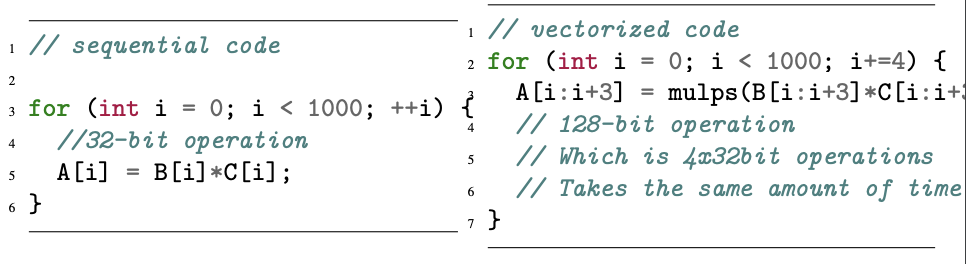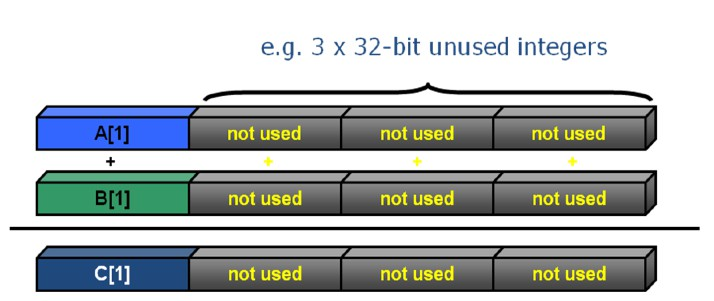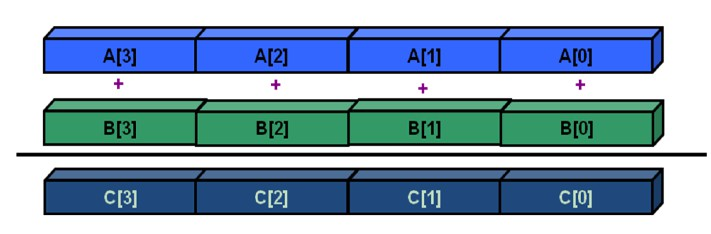# Vectorization and JAX#

Let’s now generate a following function:

$\text{signal} = \sin(2 \pi f t)$

where, $$\text{signal}$$ represents the output signal, $$\sin$$ denotes the sine function, $$\pi$$ is the mathematical constant Pi (approximately 3.14159), $$f$$ represents the frequency, and $$t$$ is the time.

import numpy as np
import time

# time step in seconds
dt = 0.005

# Frequency in Hz
f = 2.0

# Number of time steps
N = 10000000

# Numpy array containing time vector consisting of 32 bit floating point precision values
t = np.linspace(0, N * dt, N, dtype=np.float32)


## Non-vectorized for-loop#

# Non-vectorized operation that uses a Python for loop
signal2 = np.empty(N)
loop_start_time = time.time()
for i in np.arange(N):
signal2[i] = np.sin(2.0 * np.pi * f * t[i])
loop_end_time = time.time()
print(f"Vectorized Python loop time: {loop_end_time - loop_start_time:.5f} seconds")

Vectorized Python loop time: 39.63473 seconds


## Vectorized numpy#

### What is vectorization?#

Vectorization is a technique used in computer programming and numerical computing to improve the performance of code by applying operations to entire arrays or data structures instead of processing individual elements. In other words, vectorization enables the simultaneous processing of multiple data elements, which can lead to significant speedup in execution time.

This approach takes advantage of Single Instruction, Multiple Data (SIMD) hardware features present in modern processors, such as CPUs and GPUs. SIMD allows a single instruction to operate on multiple data elements concurrently, improving the overall throughput of the computation.

In the context of numerical computing, vectorization is commonly used in libraries like NumPy, JAX, and TensorFlow, where operations are applied to entire arrays or tensors at once. This eliminates the need for explicit loops in the code and allows the underlying libraries to optimize the computation using SIMD instructions or parallel execution on GPUs and TPUs.Non-vectorized for-loopVectorized iteration

Benefits of vectorization:

1. Performance: Vectorized operations can be significantly faster than their non-vectorized counterparts due to parallelism and efficient use of processor resources.

2. Code readability: Vectorized code is often more concise and easier to read than non-vectorized code, as it eliminates the need for explicit loops and focuses on high-level operations.

3. Portability: Vectorized code is more likely to benefit from performance improvements in hardware or software libraries, as it is designed to take advantage of SIMD instructions and parallelism.

However, vectorization may not be suitable for all types of problems, particularly those with complex dependencies or irregular data structures. In such cases, other optimization techniques, like parallelization or algorithmic improvements, might be more appropriate.

### Vectorization in Numpy#

Numpy is a the fundamental package for scientific computing in Python. Although Numpy is a Python package, it was not developed in Python. Rather, it is written mostly in C and consists of binary executables compiled from source code. Numpy functions are therefore generally significantly faster than the same operations performed in Python. The term “vectorized operation” refers to passing an entire Numpy array of known data type to an optimized, compiled C code. The example below shows a simple calculation of a harmonic function using vectorized operations compared with the same operation in a Python loop. The vectorized calculation is much faster.

# Vectorized operation that passes time array into Numpy sin function
vectorized_start_time = time.time()
signal1 = np.sin(2.0 * np.pi * f * t)
vectorized_end_time = time.time()
print(
f"Vectorized operation time: {vectorized_end_time - vectorized_start_time:.5f} seconds"
)
# Ratio of execution times
print(
f"Vectorized operation is {(loop_end_time - loop_start_time) / (vectorized_end_time - vectorized_start_time):.1f} times faster"
)

Vectorized operation time: 0.09802 seconds
Vectorized operation is 404.4 times faster


## Larger-domain size#

# Number of time steps
N = 100000000

# Numpy array containing time vector consisting of 32 bit floating point precision values
t = np.linspace(0, N * dt, N, dtype=np.float32)

# Vectorized operation that passes time array into Numpy sin function
vectorized_start_time = time.time()
signal1 = np.sin(2.0 * np.pi * f * t)
vectorized_end_time = time.time()
print(
f"Vectorized operation time: {vectorized_end_time - vectorized_start_time:.5f} seconds"
)

Vectorized operation time: 1.13637 seconds


## JAX#

JAX is a Python library developed by Google for optimized scientific computing:

• JAX can be considered an alternative to NumPy, as it provides a very similar interface while also offering support for GPUs and TPUs. JAX includes jax.numpy, which closely mirrors the NumPy API, making it easy for developers to transition to JAX. Most operations that can be performed with NumPy can also be performed with jax.numpy.

• JAX runs on accelerators (e.g., GPUs and TPUs) by leveraging Just-In-Time (JIT) compilation of both Python and JAX code with XLA (Accelerated Linear Algebra, a compiler) to generate optimized kernels. A kernel is a routine specifically compiled for high-throughput accelerators (e.g., GPUs and TPUs) that can be utilized by a main program. JIT compilation can be initiated using jax.jit().

• JAX offers robust support for automatic differentiation, which is particularly useful for machine learning research. Automatic differentiation can be activated with jax.grad().

• JAX promotes functional programming, as its functions are pure. Unlike NumPy arrays, JAX arrays are always immutable.

import jax.numpy as jnp
import time

# JAX array containing time vector consisting of 32 bit floating point precision values
t = jnp.linspace(0, N * dt, N, dtype=jnp.float32)

# Vectorized operation that passes time array into JAX sin function
jax_start_time = time.time()
signal1 = jnp.sin(2.0 * jnp.pi * f * t)
jax_end_time = time.time()
print(
f"JAX CPU operation time: {jax_end_time - jax_start_time:.5f} seconds"
)

JAX CPU operation time: 0.31931 seconds


### Run on GPU/TPU node#

Runtime >> Change Runtime Type to GPU.

# Vectorized operation that passes time array into JAX sin function
jax_gpu_start_time = time.time()
signal1 = jnp.sin(2.0 * jnp.pi * f * t)
jax_gpu_end_time = time.time()
print(
f"JAX GPU operation time: {jax_gpu_end_time - jax_gpu_start_time:.5f} seconds"
)

JAX GPU operation time: 0.00056 seconds